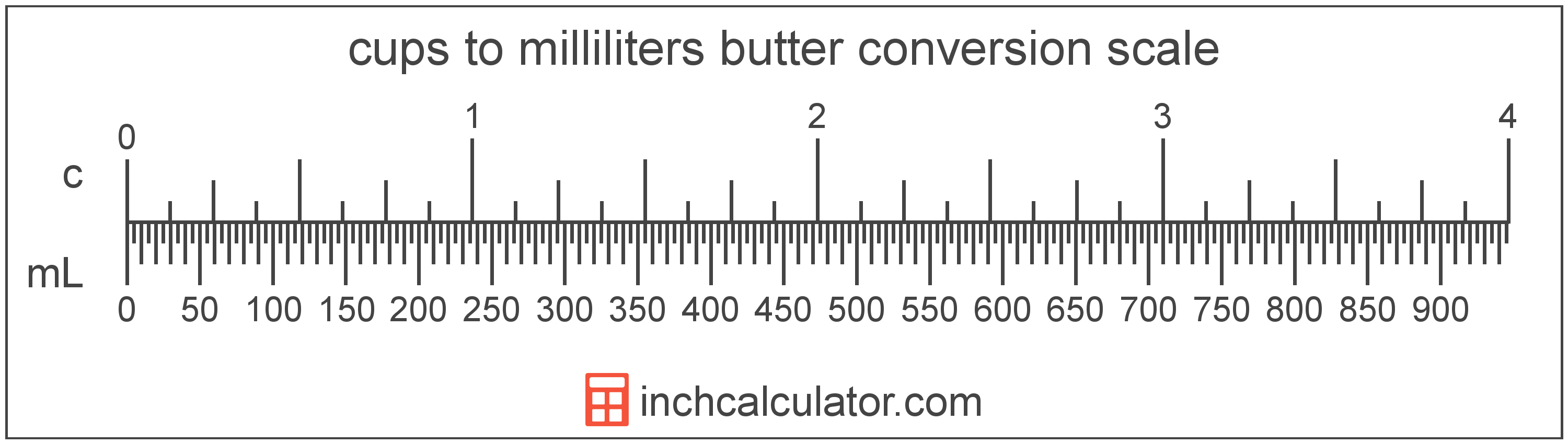# Convert Cups of Butter to Milliliters

Enter the butter in cups below to get the value converted to milliliters.

Results in Milliliters:1 c = 236.58824 mL

Do you want to convert milliliters to cups?

## How to Convert Cups to Milliliters

To convert a measurement in cups to a measurement in milliliters, multiply the butter by the following conversion ratio: 236.58824 milliliters/cup.

Since one cup of butter is equal to 236.58824 milliliters, you can use this simple formula to convert:

milliliters = cups × 236.58824

The butter in milliliters is equal to the butter in cups multiplied by 236.58824.

For example, here's how to convert 5 cups to milliliters using the formula above.
milliliters = (5 c × 236.58824) = 1,182.9412 mL### How Many Milliliters Are in a Cup of Butter?

There are 236.58824 milliliters in a cup of butter, which is why we use this value in the formula above.

1 c = 236.58824 mL

## What is a Cup?

One cup of butter is equal to 2 sticks, or 1/2 pound.

The cup is a US customary unit of volume. Cups can be abbreviated as c, and are also sometimes abbreviated as C. For example, 1 cup can be written as 1 c or 1 C.

## What is a Milliliter?

Butter is sometimes measured by volume in milliliters. One stick of butter contains about 118.3 milliliters.

The milliliter is an SI unit of volume in the metric system. A milliliter is sometimes also referred to as a millilitre. Milliliters can be abbreviated as mL; for example, 1 milliliter can be written as 1 mL.

## Cup to Milliliter Conversion Table

Table showing various cup measurements converted to milliliters.
Cups Milliliters
1 c 236.59 mL
2 c 473.18 mL
3 c 709.76 mL
4 c 946.35 mL
5 c 1,183 mL
6 c 1,420 mL
7 c 1,656 mL
8 c 1,893 mL
9 c 2,129 mL
10 c 2,366 mL
11 c 2,602 mL
12 c 2,839 mL
13 c 3,076 mL
14 c 3,312 mL
15 c 3,549 mL
16 c 3,785 mL
17 c 4,022 mL
18 c 4,259 mL
19 c 4,495 mL
20 c 4,732 mL
21 c 4,968 mL
22 c 5,205 mL
23 c 5,442 mL
24 c 5,678 mL
25 c 5,915 mL
26 c 6,151 mL
27 c 6,388 mL
28 c 6,624 mL
29 c 6,861 mL
30 c 7,098 mL
31 c 7,334 mL
32 c 7,571 mL
33 c 7,807 mL
34 c 8,044 mL
35 c 8,281 mL
36 c 8,517 mL
37 c 8,754 mL
38 c 8,990 mL
39 c 9,227 mL
40 c 9,464 mL